# 2nd PUC Maths Question Bank Chapter 12 Linear Programming Ex 12.2

Students can Download Maths Chapter 12 Linear Programming Ex 12.2 Questions and Answers, Notes Pdf, 2nd PUC Maths Question Bank with Answers helps you to revise the complete Karnataka State Board Syllabus and score more marks in your examinations.

## Karnataka 2nd PUC Maths Question Bank Chapter 12 Linear Programming Ex 12.2

### 2nd PUC Maths Linear Programming NCERT Text Book Questions and Answers Ex 12.2

Question 1.
Reshma wishes to mix two types of food P and Q in such a way that the vitamin contents of the mixture contain at least 8 units of vitamin A and 11 units of vitamin B. Food P costs ₹ 60/ kg and Food Q costs t 80/kg. Food P contains 3 units/kg of Vitamin A and 5 units / kg of Vitamin B while food Q contains 4 units/kg of Vitamin A and 2 units/kg of vitamin B. Determine the minimum cost of the mixture.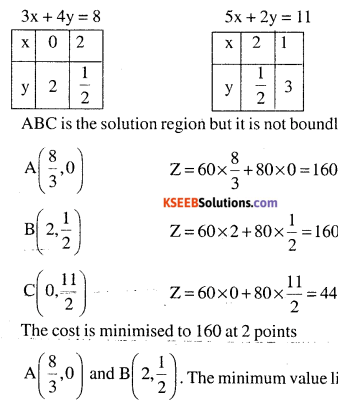Z = 60x + 80y subject to
(i) 3x + 4y ≥ 8
(ii) 5x + 2y ≥ 11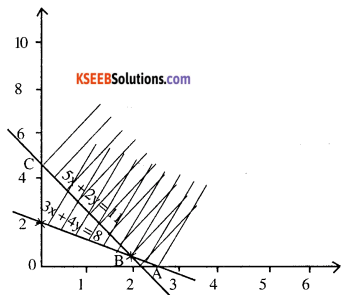$$A\left(\frac{8}{3}, 0\right) \text { and } B\left(2, \frac{1}{2}\right)$$. The minimum value lies in the line joining the points $$\left(\frac{8}{3}, 0\right) \text { and } \mathrm{B}\left(2, \frac{1}{2}\right)$$Question 2.
One kind of cake requires 200g of flour and 25g of fat, and another kind of cake requires 100g of flour and 50g of fat. Find the maximum number of cakes which can be made from 5kg of flour and 1 kg of fat assuming that there is no shortage of the other ingredients used in making the cakes.Z = x + y subject to the constraints
(i) 200 x + 100y ≤ 5000 ⇒ 2x + y <50
(ii) 25x + 50y ≤ 1000 ⇒ x + 2y < 40
(iii) x , y ≥ 0ABCD is the solution region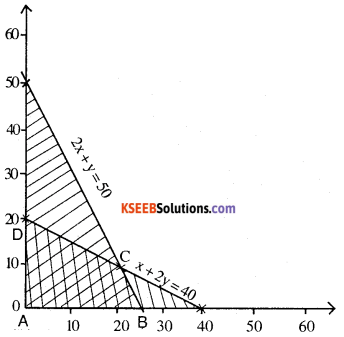A (0, 0 )  Z = 0
B (25, 0)                              Z = 25 + 0 = 25
C(20, 10)                             Z = 20+10 = 30
D (0, 20)                              Z = 0 + 20 = 20
At C (20, 10) maximum number of cakes can be made.

Question 3.
A factory makes tennis rackets and cricket bats. A tennis racket takes 1.5 hours of machine time and 3 hours of craftman’s time in its making while a cricket bat takes 3 hour of machine time and 1 hour of craftman’s time. In a day, the factory has the availability of not more than 42 hours of machine time and 24 hours of craftsman’s time.
(i) What number of rackets and bats must be made if the factory is to work at full capacity?
(ii) If the profit on a racket and on a bat is ₹ 20 and ₹ 10 respectively, find the maximuin profit of the factory when it works at full capacity.Z = x +y P = 20x +10 y
Z subject to constraints
(i) $$\frac{3}{2} x+3 y \leq 42 \quad x+2 y \leq 28$$
(ii) 3x + y ≤ 24
(iii) x ≥ 0; y ≥ 0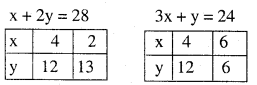ABCD is the solution region
A(0,0)   Z = 0  P = 0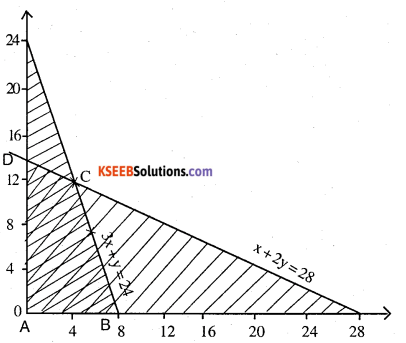ABCD is the solution region
A (0,0)   Z = 0   P = 0
B (8,0)    Z = 8    P=20 x 8 = ₹ 160
C (4. 12)  Z = 16  P = ₹ 200
D (0,14)   Z = 14  P = ₹ 140
At C (4, 12) Z is maximised to 16 and then profit is also maximised by the manufacture of 4 rackets and 12 bats.Question 4.
A manufacturer produces nuts and bolts. It takes 1 hour of work on machine A and 3 hours on machine B to , produce a package of nuts. It takes 3 hours on machine A and 1 hour on machine B to produce a package of bolts .He earns a profit of ? 17.50 per package on nuts and ? 7.00 per package on bolts. How many packages of each should be produced each day his machines for at the most 12 hours a day?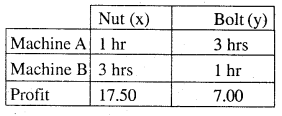$$P=\frac{35}{2} x+7 y$$
(i) x + 3y ≤ 12
(ii) 3x + y ≤ 12
(iii) x ≥0,y≥0ABCD is the solution region A(0,0) P = 0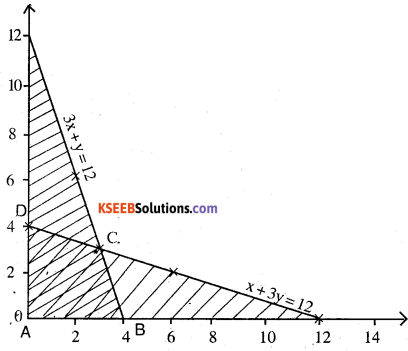A (0,0) p = 0
B (4,0)                    P = 70 ₹                                                                  .
C (3, 3)                   P = 73.5 ₹
D (0, 4)                  P = 28 ₹
At (3, 3) he can maximise his profit. By producing 3 packages of nuts and bolts each.

Question 5.
A factory manufactures two types of screws, A and B. Each type of screw requires the use of two machines, an automatic and a hand operated. It takes 4 minutes on the automatic and 6 minutes on hand operated machines to manufacture a package of screws A, while it takes 6 minutes on automatic and 3 minutes on the hand operated machines to manufacture a package of screws B. Each machine is available for at the most 4 hours on any day. The manufacturer can sell a package of screws A at a profit of ₹ 7 and screws B at a profit of ₹ 10. Assuming that he can sell all the screws he manufactures, how many packages of each type should the factory owner produce in a day in order to maximise his profit? Determine the

 Time on A(x) B(y) Total (i) automatic machine 4 min 6 min 4 hrs (ii) Hand operated machine 6 min 3 min 4 hrs (iii) Profit ₹ 7 ₹ 10

P = 7x +10y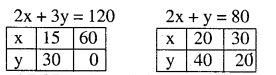(i) 4x + 6y ≤ 240 ⇒ 2x + 3y ≤120
(ii) 6x + 3y ≤ 240 ⇒ 2x + y ≤ 80
(iii) x ≥ 0 ; y ≥ 0ABCD is the solution region
A (0,0) P = 7 x 0+10 x 0 = 0
B (40, 0) P = 7 x 40+ 10 x 0 = 280 ₹
C (30, 20) P = 7 x 30+ 10 x 20 = 410 ₹
D (0, 40) P = 7 x 0+ 10 x 40 = 400 ₹
At C (30,20)P is maximised
∴ 30 types of screw A & 20 types of screw B.Question 6.
A cottage industry manufactures pedestal lamps and wooden shades, each requiring the use of a grinding/cutting machine and a sprayer. It takes 2 hours on grinding/cutting machine and 3 hours on the sprayer to manufacture a pedestal lamp. It takes 1 hour on the grinding/ cutting machine and 2 hours on the sprayer to manufacture a shade. On any day, the sprayer is available for at the most 20 hours and the grinding/cutting machine for at the most 12 hours. The profit from the sale of a lamp is ₹ 5 and that from a shade is ₹ 3. Assuming that the manufacturer can sell all the lamps and shades that he produces, how should he schedule his daily production in order to maximise his profit?P = 5x + 3y subject to the constraints
(i) 2x + y ≤ 12
(ii) 3x + 2y ≤ 20
(iii) x ≥0; y ≥0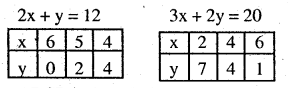ABCD is the solution region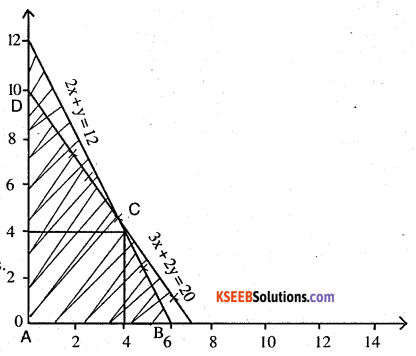A(0,0) P= 0
B(6,0) P = 5 x 6+3 x 0 = 30 ₹
C(4,4) P = 5 x 4+3 x 4 = 32 ₹
D(0,10) P = 5 x 0+3 x 10 = 30 ₹
At C (4, 4) p is maximised to  32 ₹
i.e. 4 pedestal lamps and 4 wooden shades.Question 7.
A company manufactures two types of novelty souvenirs made of plywood. Souvenirs of type A require 5 minutes each for cutting and 10 minutes each for assembling. Souvenirs of type B require 8 minutes each for cutting and 8 minutes each for assembling. There are 3 hours 20 minutes available for cutting and 4 hours for assembling. The profit is ₹ 5 each for type A and ₹ 6 each for type B souvenirs. How many souvenirs of each type should the company manufacture in order to maximise the profit?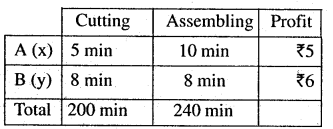P = 5x + 6y
subject to the constraints(i) 5x + 8y ≤ 200
(ii) 10x + 8y ≤ 240 ⇒ 5x + 4y ≤ 120
(iii) x ≥ 0; y ≥ 0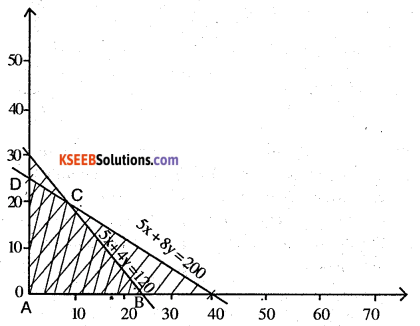A (0,0) P = 5x + 6y = 0
B (24,0) P = 5 x 24+ 6 x 0= 120 ₹
C (8,20) P = 5 x 8 + 6 x 20 = 160 ₹
D (0, 25) P = 5 x 0+ 6 x 25 = 150 ₹
At C (8, 20) P is maximised to ₹ 160
∴ 8 types of A and 2 : 6 types of B

Question 8.
A merchant plans to sell two types of personal computers a desktop model and a portable model that will cost ₹ 25000 and ₹ 40000 respectively. He estimates that the total monthly demand of computers will not exceed 250 units. Determine the number of units of each type of computers which the merchant should stock to get maximum profit if he does not want to invest more than ₹ 70 lakhs and if his profit on the desktop model is ₹ 4500 and on portable model is ₹ 5000.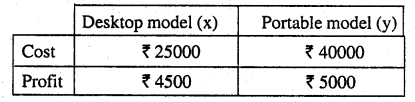P = 4500 x + 5000 y subject to
(i) x + y ≤ 250
(ii) 25000 x + 40000 y ≤ 7000000
5x + 8y ≤ 1400
x ≥ 0; y ≥ 0ABCD is the solution region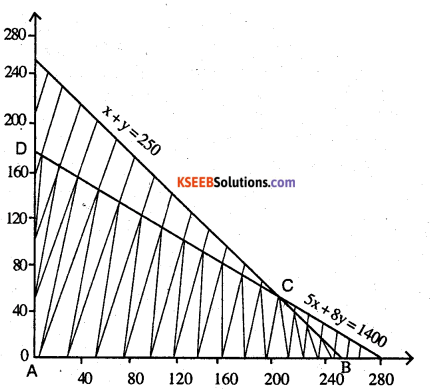A (0,0), P = 4500 x + 5000 y = 0
B (250,0), P = 4500 x 250 = ₹ 1125000
C (200,50), P = 4500 x 200 + 5000 x 50 = ₹ 1150000
D (0,175), P = 5000 x 175 = ₹ 875000
∴ The profit is maximised at C (200,50) ₹ 1150000Question 9.
A diet is to contain at least 80 units of vitamin A and 100 units of minerals. Two foods F1 and F2 are available. Food F2 costs ₹ 4 per unit food and F2 costs ₹ 6 per unit. One unit of food F1 contains 3 units of vitamin A and 4 units of minerals. One unit of food F2 contains 6 units of vitamin A and 3 units of minerals. Formulate this as a linear programming problem. Find the minimum cost for diet that consists of mixture of these two foods and also meets the minimal nutritional requirements.Minimize Z = 4x + 6y
(i) 3x + 6y ≥ 80
(ii) 4x + 3y ≥100
(iii) x ≥ 0 ; y ≥ 0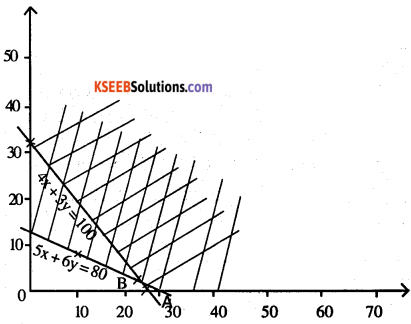Question 10.
There are two types of fertilisers F1 and F2 .F1 consists of 10% nitrogen and 6% phosphoric acid and F2 consists of 5 % nitrogen and 10% phosphoric acid. After testing the soil conditions, a farmer finds that she needs atleast 14 kg of nitrogen and 14 kg of phosphoric acid for her crop. If F1 costs ₹ 6/kg and F2 costs ₹ 5/kg, determine how much of each type of fertiliser should be used so that nutrient requirements are met at a minimum cost. What is the minimum cost?B (100,80) Z=1000
C (0,280) Z = 1400
Cost is minimum at B (100, 80)
∴ F1 = 100   F2 = 80Question 11.
The corner points of the feasible region determined by the following system of linear inequalities:
2x + y ≤ 10, x + 3y ≤ 15, x, y ≥ 0 are (0, 0), (5, 0), (3, 4) and (0, 5). Let Z = px + qy, where p, q >0 . Condition on p and q so that the maximum of Z occurs at both (3, 4) and (0, 5) is.
(A) p = q
(B) P = 2q
(C) p = 3q
(D) q = 3p